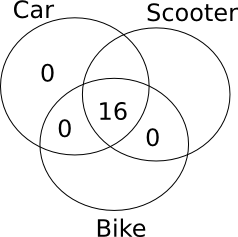# Venn Diagram Questions For CAT PDF Set-2

0
15167

Venn Diagrams are an essential component of the Logical Reasoning section and are used to visualize relationships between sets and determine their properties. Solving questions on Venn Diagrams is a great way to improve your understanding and proficiency in this area. This set of questions includes a variety of important practice questions on Venn Diagrams, each of which comes with a detailed solution. By solving these questions, you can test your understanding of Venn Diagrams and improve your ability to solve problems accurately and efficiently. Click on the below link to download the Venn Diagram Questions and Answers for CAT exam PDF set-2 with solutions.

Question 1:  In a school, 150 students have to opt for at least one subject out of English, Physics and Mathematics. If 71 students opted for English, 98 students opted for Maths, 68 students opted for Physics and 14 students opted for all the three subjects, find the number of students who opted for exactly two subjects.

a) 49
b) 59
c) 39
d) 69

Question 2: In an exam conducted for 38 students of class XII 15 passed in Biology and 25 passed in Chemistry. If atleast 1 student failed in both the subjects,find the maximum number of students who passed in only Biology ?

a) 12
b) 15
c) 10
d) 13

Question 3: In a locality, residents read at least one of the three newspapers – Hindu, Times and Express. 60% read Hindu, 80% read Times and 55% read Express. What can be the minimum and maximum percentage of residents who read exactly two newspapers?

a) 2.5%, 92.5%
b) 5%, 95%
c) 10%, 90%
d) 0%, 95%

Question 4: Out of 60 families living in a building, all those families which own a car own a scooter as well. No family has just a scooter and a bike. 16 families have both a car and a bike. Every family owns at least one type of vehicle and the number of families that own exactly one type of vehicle is more than the number of families that own more than one type of vehicle. What is the sum of the maximum and minimum number of families that own only a bike?

a) 24
b) 34
c) 44
d) 54

Question 5: 207 people who attend “Bold Gym” in Kondapur take four types juices Apple, Orange, Pomegranate and Mango. There are a few people who do not take any of the juices. It is known that for every person in the Gym who takes atleast ‘N’ types of juices there are 2 persons who take atleast ‘N-1’ juices for N = 2, 3 and 4. If the number of people who take all four types of juices is equal to the number of people who do not take any juice at all, what is the number of people who take exactly 2 types of juices?

a) 23
b) 46
c) 69
d) 92

Let A, B and C be the sets of students who opted for English, Physics and Maths respectively.

We know that the total number of students is 150 which can be represented by the union of the three sets A, B and C i.e. $AUBUC$

$AUBUC = A+B+C-A\bigcap B-B\bigcap C-C\bigcap A+A\bigcap B\bigcap C$

150 =$71+98+68-A\bigcap B-B\bigcap C-C\bigcap A+A\bigcap B\bigcap C+14$

$A\bigcap B+B\bigcap C+C\bigcap A = 251-150 = 101$

But this $A\bigcap B+B\bigcap C+C\bigcap A$ also has students who opted for all 3 subjects and they have been counted 3 times. So we have to subtract them i.e. 14 students three times.

Hence the number of students who chose exactly 2 subjects = 101-(14*3) = 59From the Venn diagram , a + b = 15 – (1)

b + c = 25 – (2)

a + b + c + n = 38 – (3)

(3) – (2) – (1) = b – n = 2

as n\geq≥1 the minimum value of b is 3.

From (1) the maximum value of a is 12

Let ‘a’ be the percentage of residents who read only one newspaper. Let ‘b’ be the percentage of residents who read exactly two newspapers. Let ‘c’ be the percentage of residents who read all three newspapers.

a + b + c = 100
a + 2b + 3c = 60 + 80 + 55 = 195
=> b + 2c = 95
To find the maximum percentage of residents who read exactly two newspapers, ‘b’ has to be maximum and ‘c’ has to be minimum. If c = 0, then b = 95, in which case a = 5.

To find the minimum percentage of residents who read exactly two newspapers, ‘b’ has to be minimum. Since b + 2c = 95, if b = 0, c = 47.5, in which case a = 52.5

So, the minimum percentage is 0% and the maximum percentage is 95%

From the information given in the question, the following Venn Diagram can be constructed:So, in order to maximize the number of families that own only a bike, we can put the remaining 44 families in ‘only bike’ region. Similarly, in order to minimize the number of families that own only a bike, we can put the remaining 44 families in ‘only scooter’ region.
So, the maximum number of families that own only a bike is 44 and the minimum number of families that own only a bike is 0.
So, sum = 44 + 0 = 44